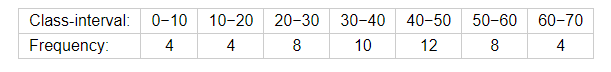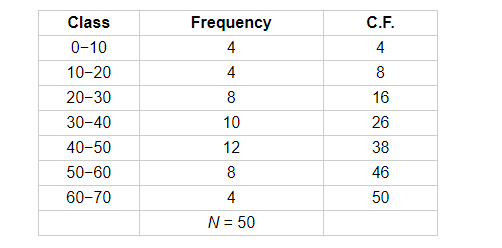# Write the median class of the following distribution:

Question:

Write the median class of the following distribution:Solution:

Consider the following distribution table.Here,

$N=50$

$\frac{N}{2}=25$

The cumulative frequency just greater than 25 is 26.

So, the median class is 30−40.